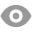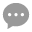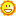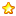# 底部放量上涨测试和代码版本汇总173 |3 |桥博士 | 2020-4-2 10:41:00 | 显示全部楼层 |阅读模式
 底部放量上涨定义与测试版本一（20200402） 1) 定义底部放量上涨的具体形态；A. 形态条件1 文字描述：价格已连续下跌一段时间，并不断创新低 量化定义：当日价格最低价创了10日内最低； B. 形态条件2 文字描述：当日价格突然上涨 量化定义：当日收盘价大于前一天的最高价； C. 形态条件3 文字描述：当日出现放量 量化定义：当日成交量大于过去1日的成交量； 2) 定义测试的买卖逻辑：A. 测试的买入条件：出现底部放量形态，以收盘价买入；B. 测试的卖出条件：买入后第二天，以收盘价卖出 3) 定义测试的品种和时间：测试品种：上证指数 测试周期：2000-02-01 到 2020-01-31(详细测试数据请看本帖3楼)

 版本一 测试一代码：（P=1，N=10，D=1） //开仓资金 LOTS:=INTPART(8000000/(C*MARGIN*UNIT+FEE));//计算开仓手数 FL:=V>REF(V,P);//放量定义 DB:=L=LLV(L,N);//定义底部：创前10日新低 SZ:=CLOSE>REF(HIGH,D);//定义上涨 //定义买入卖出 DB AND FL AND SZ,BK(LOTS); BARSBK=1,SP(BKVOL);

 版本一 测试二代码：（P=2, N=10, D=1） //开仓资金 LOTS:=INTPART(8000000/(C*MARGIN*UNIT+FEE));//计算开仓手数 FL:=V>REF(V,P);//放量定义 DB:=CREF(HIGH,D); //定义买入卖出 DB AND FL AND SZ,BK(LOTS); BARSBK=1,SP(BKVOL);

 版本一 测试三代码：（P=1，N=10，D=1，L1=6，L2=24） //开仓资金 LOTS:=INTPART(8000000/(C*MARGIN*UNIT+FEE));//计算开仓手数 //定义BIAS BIAS1:=(CLOSE-MA(CLOSE,L1))/MA(CLOSE,L1)*100;//计算短周期BIAS BIAS2:=(CLOSE-MA(CLOSE,L2))/MA(CLOSE,L2)*100;//计算长周期BIAS //定义BIAS买入条件 BIASBK:=BIAS1>BIAS2; FL:=V>REF(V,P);//放量定义 DB:=L=LLV(L,N);//创前10日新低 SZ:=CLOSE>REF(HIGH,1); //定义买入卖出 DB AND FL AND SZ AND BIASBK,BK(LOTS); BARSBK=D,SP(BKVOL);

 您需要登录后才可以回帖 登录 | 立即注册 本版积分规则 回帖后跳转到最后一页132主题 1679积分1679